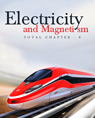Click to Chat

1800-1023-196

+91-120-4616500

CART 0

• 0

MY CART (5)

Use Coupon: CART20 and get 20% off on all online Study Material

ITEM
DETAILS
MRP
DISCOUNT
FINAL PRICE
Total Price: Rs.

There are no items in this cart.
Continue Shopping
`        A copper wire of radius 0.1 mm and resistance 1 kΩ is connected across a power supply of 20 V. (a) How many electrons are transferred per second between the supply and the wire at one end? (b) Write down the current density in the wire.`
5 years ago

```							Sol. r = 0.1 mm = 10^-4
R = 1 KΩ = 10^3 Ω, V = 20 V
a) No.of electrons transferred
i = V/R = 20/10^3 = 20  * 10^-3 = 2 * 10^-2 A
q = i t = 2 * 10^-2 * 1 = 2 * 10^-2 C.
No. of electron transferred = 2 * 10^-2/1.6 * 10^-19 = 2 * 10^-17/1.6 = 1.25 * 10^17.
b)Current density of wire
= i/A = 2 * 10^-2/π * 10^10^-8 = 2/3.14 * 10^+6
0.6369 * 10^+6 = 6.37 * 10^5 A/m^2.

```
5 years ago
Think You Can Provide A Better Answer ?

## Other Related Questions on Electromagnetic Induction

View all Questions »### Course Features

• 731 Video Lectures
• Revision Notes
• Previous Year Papers
• Mind Map
• Study Planner
• NCERT Solutions
• Discussion Forum
• Test paper with Video Solution### Course Features

• 57 Video Lectures
• Revision Notes
• Test paper with Video Solution
• Mind Map
• Study Planner
• NCERT Solutions
• Discussion Forum
• Previous Year Exam Questions